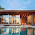## Thursday, 26 January 2017

### Processing GeoData Files using QGis functions in Matlab

It's always really useful to streamline your workflow, so that the processes can be seamless and efficient.

In that regard, I create scenarios for my crowd application that take in Shape files and rasterised GeoTiff files to specify a scenario. The GeoTiff files are obtained by rasterising the shape files using the 'rasterize' tool within QGis.QGis Rasterize tool
Sometimes, the conversion from vector to raster is not as desired, where some pixel values are a pixel out from where they should be, and therefore, requires some editing. This can be done in Photoshop, however, Photoshop doesn't save the geodata from the GeoTiff file. A tool such as FWTools is needed in order to extract the geographical metadata before editing, and then appending it to the edited Tiff file. For completeness, here is what needs to be done, within the FWTools shell.
`````` C:\> listgeo -no_norm original.tif > metadata.geo
``````
Once edited, copy the metadata to the edited tiff file:
`````` C:\> geotifcp -g metadata.geo edited.tif output.tif
``````
This process becomes pretty tedious over time. A better solution is needed.

Set up the QGis environment within Matlab

Underneath the hood, QGis uses the Gdal library. Python scripts can be written within QGis to streamline the process, however, it's not possible to edit the GeoTiff file within QGis. Therefore, it would then require me to use the FWTools method, as described above.

On the other hand, Matlab already has a GeoTiff reader and writer that we can use to edit the GeoTiff file if required after converting the Shape file to GeoTiff. A simple way to do this is to write a script to first set up the QGis environment within Matlab, as follows:

`````` QGISTOOLS_DIR='C:\Program Files\QGIS 2.18';
setenv('QGISTOOLS_DIR', QGISTOOLS_DIR);
setenv('PATH', [QGISTOOLS_DIR '\bin;' getenv('PATH')]);
``````

Now, any QGis functions can be called using Matlab's symbol for calling system functions ('!').

Write a Matlab script to use QGis functions

After setting up the QGis environment, we can now call the QGis functions from within Matlab. We may also want to pass user input to the QGis functions. In order to convert the user input into a Matlab expression that is passed to QGis, we can use the 'eval' function of Matlab

Here, the user input for 'layer', 'input' and 'output' is passed onto the QGis 'gdal_rasterize' function. The code is below:

`````` % [img, R, info] = rasterise(layer, input, output)
%
% This function takes a layer and shape file as input
%
% It outputs a GeoTiff file using the GET attribute set in the shape file
% The GET attribute is specified in the scenario specification work flow
% document
%
% The function also optionally reads the image data into img, the spatial
% referencing object into R, and the geotiff info into info

function [img, R, info] = rasteriseShp(layer, input, output)

% Call the QGis Tools Environment setup script for this session
QGisToolsSetup

eval(['!gdal_rasterize -a GET -tr 1.0 1.0 -l ' layer ' "' input '" "' output '"']);

info = geotiffinfo(output);

end
``````

I can now edit the 'img' data matrix if necessary, and write it out using Matlab's own 'geotiffwrite' function, that would retain the geographical metadata.
`````` geotiffwrite('output.tif', img, R);
``````
Similarly, this process can be used for any QGis function within Matlab once the environment is setup. I am also using QGis functions instead of FWTools functions now, as QGis has a later version of 'gdal_rasterize' that I needed.

Setting up FWTools within Matlab

Whilst we are on the topic of setting up environments within Matlab, a quick mention that FWTools can also be set up and used within Matlab in a similar way. An example set up code can be found here, and I will repeat it below for the latest version:

`````` % Hamish & Cerys, 9 May 2008
% This script is public domain code
% setup environment for FWTools

FWTOOLS_DIR='C:\Program Files (x86)\FWTools2.4.7';
setenv('FWTOOLS_DIR', FWTOOLS_DIR);
setenv('PATH', [FWTOOLS_DIR '\bin;' FWTOOLS_DIR '\python;' getenv('PATH')]);
setenv('PYTHONPATH', [FWTOOLS_DIR '\pymod']);
setenv('PROJ_LIB', [FWTOOLS_DIR '\proj_lib']);
setenv('GEOTIFF_CSV', [FWTOOLS_DIR '\data']);
setenv('GDAL_DATA', [FWTOOLS_DIR '\data']);
setenv('GDAL_DRIVER_PATH', [FWTOOLS_DIR '\gdal_plugins']);
``````

P.S If you need an easy way to format source code within blogger, you can find a link to a good source code formatter here.

1.Really Awesome………very nice post. You are Expert in your profession. It's a very interesting in a post. I appreciate you keep do a posting..
Wardrobes Dublin
Sliding wardrobe Cork
Fitted wardrobe
fitted wardrobes dublin
Half Height Wardrobes

2.3.